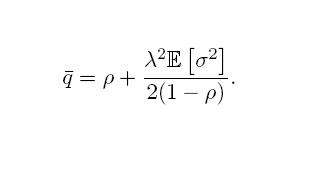## Jan 27, 2006

### Exercise 4 - Simulation of a M/D/1 queue

Consider a simple network composed of only two nodes (S and D), and one link connecting them. The link connecting the two nodes has a speed of 1Mbps, a propagation delay of 100 ms, and a DropTail buffer at its input of very large size.

\$ns duplex-link \$S \$D 1Mb 100ms DropTail
\$ns queue-limit \$S \$D 10000

We run on node S a Poisson source of traffic. The destination of the packets being node D. Let lamda be the intensity of the Poisson traffic in terms of packets/s. Take lamda = 1200 packets/s and set the packet size of the Poisson source to a fixed value 100 bytes.

Write the code of a simulation of this scenario, then run the simulation for 1000 seconds. The Poisson source can be created as follows:

set udp [new Agent/UDP]
\$ns attach-agent \$S \$udp
set null [new Agent/Null]
\$ns attach-agent \$D \$null
\$ns connect \$udp \$null
set poisson [new Application/Traffic/Poisson]
\$poisson attach-agent \$udp
\$ns at 0 "\$poisson start"

Don’t forget the ns run !

Verify the load of the queue. What is the expected load? You get the load from the simulation by computing the total number of packets that leave the queue, then by dividing it by the total number of packets that would leave the queue if the link was busy all time. Use the queue monitor object to obtain the total number of packets that leave the queue.

set monitor [\$ns monitor-queue \$S \$D stdout]

We focus on the computation of the average queue length. Compute theoretically this average value. Recall that the average queue length in a M/G/1 queue is equal toThis average queue length includes the packet in the server (physically, the packet being transmitted). The Queue-Monitor object gives the number of packets waiting in the queue, without counting the packet in the server. You will notice that there is a difference P between the theoretical value and the measured value. P is the average number of packets occupying the server of the queue.

Now validate this average value using your simulation. You need to write a function that samples the queue length many times during the simulation, then you compute the average over all the samples you obtain.

proc queueLength {sum number}{
global ns monitor
set time 0.1
set len [\$monitor set pkts_]
set now [\$ns now]
set sum [expr \$sum+\$len]
set number [expr \$number+1]
puts "[expr 1.0*\$sum/\$number]"
\$ns at [expr \$now+\$time] "queueLength \$sum \$number"}
\$ns at 0 "queueLength 0 0"

As a final exercise on this scenario, write in a file the queue size in packets (on two columns, the first one for time, the second one for queue size), and plot this queue size versus time using gnuplot.

*** for generation of random inter-arrival time and packet sizes

such example can be used :

set lambda 30.0
set mu 33.0
set InterArrivalTime [new RandomVariable/Exponential]
\$InterArrivalTime set avg_ [expr 1/\$lambda]
set pktSize [new RandomVariable/Exponential]
\$pktSize set avg_ [expr 100000.0/(8*\$mu)]

#### 1 comment:Anonymous said...

Hello,
I'm interested in this exercise... Have you got the solution?